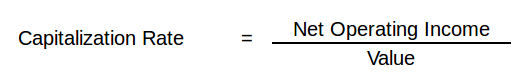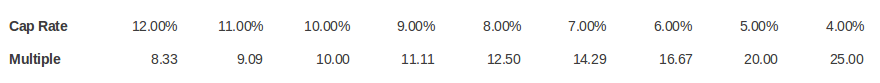# Cap Rates

The capitalization rate is a fundamental concept in the commercial real estate industry. Yet, it is often misunderstood and sometimes incorrectly used. This post will take a deep dive into the concept of the cap rate, and also clear up some common misconceptions.

Definition

What is a cap rate? The capitalization rate, often just called the cap rate, is the ratio of Net Operating Income (NOI) to property asset value. So, for example, if a property recently sold for \$1,000,000 and had an NOI of \$100,000, then the cap rate would be \$100,000/\$1,000,000, or 10%.Cap Rate Example

Let’s take an example of how a cap rate is commonly used. Suppose we are researching the recent sale of a Class A  office building with a stabilized Net Operating Income (NOI) of \$1,000,000, and a sale price of \$17,000,000. In the commercial real estate industry, it is common to say that this property sold at a 5.8% cap rate.

Intuition Behind the Cap Rate

What is the cap rate actually telling you?  One way to think about the cap rate intuitively is that it represents the percentage return an investor would receive on an all cash purchase.  In the above example, assuming the real estate proforma is accurate, an all cash investment of \$17,000,000 would produce an annual return on investment of 5.8%. Another way to think about the cap rate is that it’s just the inverse of the price/earnings multiple.  Consider the following chart:As shown above, cap rates and price/earnings multiples are inversely related.  In other words, as the cap rate goes up, the valuation multiple goes down.

When, and When Not, to Use a Cap Rate

The cap rate is a very common and useful ratio in the commercial real estate industry and it can be helpful in several scenarios.  For example, it can and often is used to quickly size up an acquisition relative to other potential investment properties.  A 5% cap rate acquisition versus a 10% cap rate acquisition for a similar property in a similar location should immediately tell you that one property has a higher risk premium than the other.

Another way cap rates can be helpful is when they form a trend.  If you’re looking at cap rate trends over the past few years in a particular sub-market then the trend can give you an indication of where that market is headed.  For instance, if cap rates are compressing that means values are being bid up and a market is heating up. Where are values likely to go next year?  Looking at historical cap rate data can quickly give you insight into the direction of valuations.

While cap rates are useful for quick back of the envelope calculations, it is important to note when cap rates should not be used. When properly applied to a stabilized Net Operating Income (NOI) projection, the simple cap rate can produce a valuation approximately equal to what could be generated using a more complex discounted cash flow (DCF) analysis. However, if the property’s net operating income stream is complex and irregular, with substantial variations in cash flow, only a full discounted cash flow analysis will yield a credible and reliable valuation.

Components of the Cap Rate

What are the components of the cap rate and how can they be determined?  One way to think about the cap rate is that it’s a function of the risk free rate of return plus some risk premium.  In finance, the risk free rate is the theoretical rate of return of an investment with no risk of financial loss.  Of course in practice all investments carry even a small amount of risk. However, because U.S. bonds are considered to be very safe, the interest rate on a U.S. treasury bond is normally used as the risk-free rate. How can we use this concept to determine cap rates?

Suppose you have \$10,000,000 to invest and 10-year treasury bonds are yielding 3% annually. This means you could invest all \$10,000,000 into treasuries, considered a very safe investment, and spend your days at the beach collecting checks. What if you were presented with an opportunity to sell your treasuries and instead invest in a Class A office building with multiple tenants? A quick way to evaluate this potential investment property relative to your safe treasury investment is to compare the cap rate to the yield on the treasury bonds.

Suppose the acquisition cap rate on the investment property was 5%.  This means that the risk premium over the risk free rate is 2%.  This 2% risk premium reflects all of the additional risk you assume over and above the risk free treasuries, which takes into account factors such as:

• Age of the property.
• Credit worthiness of the tenants.
• Diversity of the tenants.
• Length of tenant leases in place.
• Broader supply and demand fundamentals in the market for this particular asset class.
• Underlying economic fundamentals of the region including population growth, employment growth, and inventory of comparable space on the market.

When you take all of these items and break them out, it’s easy to see their relationship to the risk free rate and the overall cap rate. It’s important to note that the actual percentages of each risk factor of a cap rate and ultimately the cap rate itself are subjective and depend on your own business judgement and experience.

Is cashing in your treasuries and investing in an office building at a 5% acquisition cap rate a good decision?  This of course depends on how risk averse you are.  An extra 2% yield on your investment may or may not be worth the additional risk inherent in the property. Perhaps you are able to secure favorable financing terms and using this leverage you could increase your return from 5% to 8%.  If you a more aggressive investor this might be appealing to you.  On the other hand, you might want the safety and security that treasuries provide, and a 3% yield is adequate compensation in exchange for this downside protection.

Band of Investment Method

The above risk free rate approach is not the only way to think about cap rates.  Another popular alternative approach to calculating the cap rate is to use the band of investment method.  This approach takes into account the return to both the lender and the equity investors in a deal. The band of investment formula is simply a weighted average of the return on debt and the required return on equity.  For example, suppose we can secure a loan at an  80% Loan to Value (LTV), amortized over 20 years at 6%.  This results in a mortgage constant of 0.0859.  Further suppose that the required return on equity is 15%.  This would result in a weighted average cap rate calculation of 9.87% (80%*8.59% + 20%*15%).

The Gordon Model

One other approach to calculating the cap rate worth mentioning is the Gordon Model. If you expect NOI to grow each year at some constant rate, then the Gordon Model can turn this constantly growing stream of cash flows into a simple cap rate approximation.  The Gordon Model is a concept traditionally used in finance to value a stock with dividend growth:This formula solves for Value, given cash flow (CF), the discount rate (k), and a constant growth rate (g).  From the definition of the cap rate we know that Value = NOI/Cap. This means that the cap rate can be broken down into two components, k-g.  That is, the cap rate is simply the discount rate minus the growth rate.

How can we use this? Suppose we are looking at a building with an NOI of \$100,000 and in our analysis we expect that the NOI will increase by 1% annually.  How can we determine the appropriate cap rate to use?  Using the Gordon Model, we can simply take our discount rate and subtract out the annual growth rate.  If our discount rate (usually the investor’s required rate of return) is 10%, then the appropriate cap rate to use in this example would be 9%, resulting in a valuation of \$1,111,111.

The Gordon Model is a useful concept to know when evaluating properties with growing cash flows.  However, it’s not a one-size fits all solution and has several built in limitations.  For example, what if the growth rate equals the discount rate?  This would yield an infinite value, which of course in nonsensical. Alternatively, when the growth rate exceeds the discount rate, then the Gordon Model yields a negative valuation which is also a nonsensical result.

These built-in limitations don’t render the Gordon Model useless, but you do need to be aware of them.  Always make sure you understand the assumptions you are making in an analysis and whether they are reasonable or not.